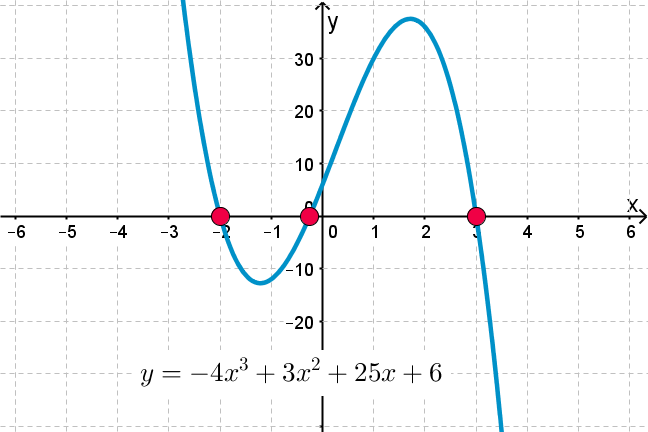## Want to keep learning?

This content is taken from the UNSW Sydney's online course, Maths for Humans: Linear, Quadratic & Inverse Relations. Join the course to learn more.
4.5

## UNSW SydneyThree zeroes hath this cubic

# Cubic polynomials and their roots

Just as for quadratic functions, knowing the zeroes of a cubic makes graphing it much simpler. Typically a cubic function will have three zeroes or one zero, at least approximately, depending on the position of the curve. Finding these zeroes, however, is much more of a challenge. In fact this challenge was a historical highlight of 16th century mathematics.

In this step we will

• see how Descartes’ factor theorem applies to cubic functions

• review how to find zeroes of a cubic in special situations.

## Zeroes of a cubic polynomial

One simple way of cooking up a cubic polynomial is just to take a product of linear factors, for example

$\Large{y=(x-4)(x-1)(x+2).}$

We hope that you can all expand the right-hand side to get

$\Large{y=x^3-3x^2-6x+8.}$

The graph of this function is shown hereNote that it does pass through the points $$\normalsize{[4,0]}$$, $$\normalsize{[1,0]}$$ and $$\normalsize{[-2,0]}$$, as it should by Descartes’ Factor theorem.

## Factorising simple cubics

Here is a simple cubic polynomial that has been chosen to have a nice factorisation: $$\normalsize{f(x)=x^3-7x+6}.$$Let us note that the curve passes through the points $$\normalsize{[1,0]}$$, $$\normalsize{[2,0]}$$ and $$\normalsize{[-3,0]}$$. This corresponds to the fact that $$\normalsize{f(1)=f(2)=f(-3)=0}$$. We say that $$\normalsize{1}$$, $$\normalsize{2}$$ and $$\normalsize{-3}$$ are the zeroes or roots of $$\normalsize{f(x)}$$.

The zeroes of a polynomial $$\normalsize{f(x)}$$ have a particular importance: from Descartes’ theorem if a polynomial $$\normalsize{p(x)}$$ has a zero $$\normalsize{r}$$, that is $$\normalsize{p(r)=0}$$, then it follows that $$\normalsize{(x-r)}$$ is a factor of $$\normalsize{p}$$.

In our example, since $$\normalsize{f}$$ has zeroes $$\normalsize{1}$$, $$\normalsize{2}$$ and $$\normalsize{-3}$$, we know that $$\normalsize{f(x)}$$ has factors $$\normalsize{(x-1)}$$, $$\normalsize{(x-2)}$$ and $$\normalsize{(x+3)}$$. And indeed

$\Large{f(x)=(x-1)(x-2)(x+3)}.$

So in practice trial and error may be used when looking for possible zeroes by hand. It is a good guess to try factors of the constant term if the polynomial is monic (that means that the highest degree coefficient is $$\normalsize{1}$$). Of course using a computer is much simpler: our electronic friends are particular adept at this kind of work!

Q1 (M): Try to factor $$\normalsize{p(x)=x^3-3x^2+4x-70}$$.

If you made progress with this factorisation, then you should find a factor of $$\normalsize (x^2+2x+14)$$ appearing, and you will find it resists further attempts to factor it. Perhaps it doesn’t factor, but how would we know that the quadratic term does not factor further?

One way is to keep trying the various possible factors, but a better way is to use the quadratic formula. The discriminant of this quadratic is $$\normalsize{b^2-4ac=2^2-4(1)(14)=-39}$$ which is not a square, so there is no rational factorisation. We say that $$\normalsize{x^2+2x+14}$$ is irreducible (over the rational numbers) and leave it at that.

Q2 (M): Find zeroes and factorisations of the following cubics:

a) $$\normalsize{g(x)= x^3-4x}$$

b) $$\normalsize{h(x)=x^3+4x^2-x-4}$$

c) $$\normalsize{k(x)= 2x^3-7x^2-14x-5}$$

Knowing the factorisation of a cubic makes it easier to graph, since we know where it crosses the $$\normalsize{x}$$-axis.

## Tartaglia, Cardano and factoring general cubics

Suppose someone gave you the cubic function

$\Large{y=(x-7)(x-53)(x+13)= x^3-47x^2-409x+4823}$

and asked you to factor it. Not knowing the left hand side of the equation, it might take some work to find the factors. But what if the cubic does not factor nicely into factors? Just by changing the cubic a little to

$\Large{y= x^3-47x^2-409x+4822}$

makes things vastly more complicated! This is just the kind of challenge that 16th century mathematicians like G. Cardano (1501-1576) and N. Tartaglia (1500-1557) gave each other.

During one of these challenges, Tartaglia discovered a general formula for solving cubics which extends the much more familiar quadratic formula. His formula is quite remarkable in that it requires complex numbers in an essential way, and also both square roots and cube roots. However it is not for the faint-hearted, and it is fair to say that these days it is rarely used.Girolamo Cardano, By Wellcome Library, London, CC BY 4.0, via Wikimedia Commons

The story of how Cardano (pictured) stole the credit for this formula from Tartaglia is one of the most juicy episodes in the history of mathematics. For this story and other historical information on cubics, check out Norman’s History of Mathematics lectures on his YouTube channel.

Tartaglia’s cubic formula is workable if your example has been chosen carefully to have linear factors in the first place. But a random cubic does not have such a property, and so most cubics can in fact only be approximately factored.

A1. Let’s look at this problem in some detail. To factor $$\normalsize{p(x)=x^3-3x^2+4x-70}$$ one should look for zeroes which are factors of $$\normalsize{70}$$, which are built from $$\normalsize{2,5}$$ and $$\normalsize{7}$$, and we should not forget about negative possible factors. Now with a bit of trial and error you can find that $$\normalsize{p(5)=0}$$, so by Descartes’ theorem we know that $$\normalsize{(x-5)}$$ is a factor.

After that we want to actually divide $$\normalsize{p(x)}$$ by $$\normalsize{(x-5)}$$. This requires polynomial long division. If you have forgotten how to do long division, you will be happy to learn that polynomial long division is generally simpler than that with numbers.

By looking at successive powers of x, we sequentially determine that the other factor must be $$\normalsize{x^2+2x+14}$$. Please try it! The final factoring is

$\Large{p(x)=(x-5)(x^2+2x+14)}.$

A2.

a) We have $$\normalsize{g(x)= x^3-4x=x(x-2)(x+2)}$$.

b) We have $$\normalsize{h(x)=x^3+4x^2-x-4=(x-1)(x+1)(x+4)}$$.

c) We have $$\normalsize{k(x)= 2x^3-7x^2-14x-5=(x-5)(x+1)(2x+1)}$$.